## 螢幕截圖

•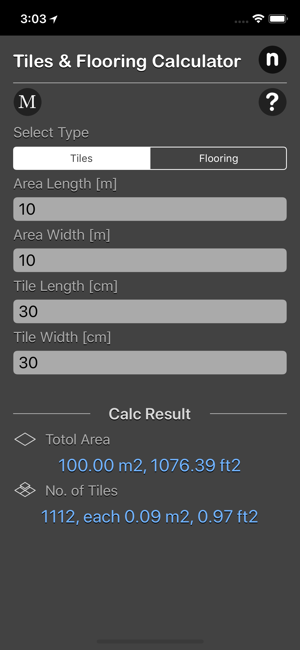•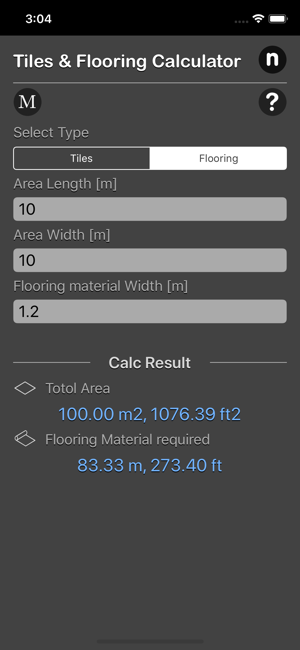•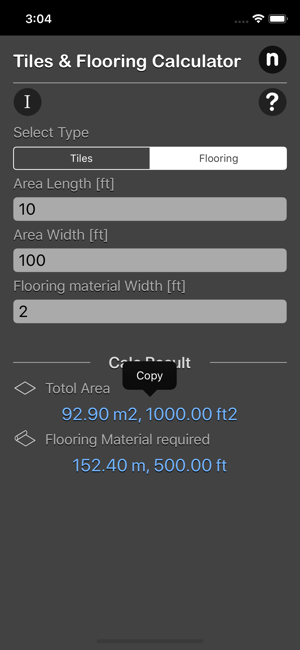•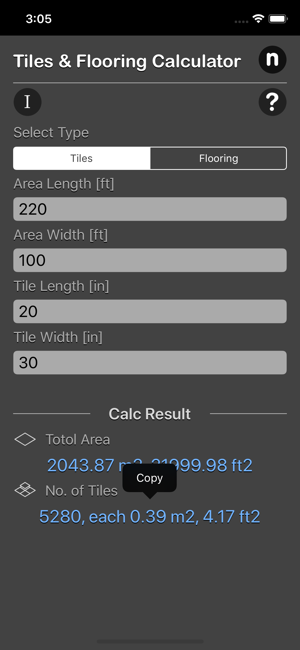•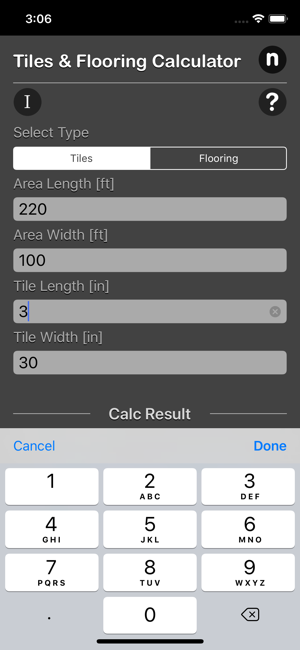## 簡介

Tiles and Flooring Calculator allows you to calculate how many tiles you need to cover a simple rectangular area. Makes it easy for you to easily estimate the number of tiles or flooring material required for your project be it tiling your roof, walls or floor.

Features:
- Works both in metric and imperial units.
- Works for both rectangular and square tiles.
- Output total area, tile number plus tiles dimension in calculation.

Carpet/ Laminate Estimation
Given the area of floor and width of vinyl roll, it is easy to determine the length of flooring material required to cover the floor. Simply divide the floor area by the width of the flooring material used.

Tile Calculation Formula
The total number of tiles can be calculated by dividing the area of the surface to be covered by the area of a single tile. Note that the units of the two area should be the same.

Example calculation: How many 30cm x 30cm tiles do i need for a floor area of 100 m2?
Ans. Number of tiles required: 1112

*This is a universal app that work for both iPhone and iPad.

Thanks for your support and do visit nitrio.com for more apps for your iOS devices.

Tan Chia Ling

16.3 MB

4+

HK\$ 23.00

## 支援

•